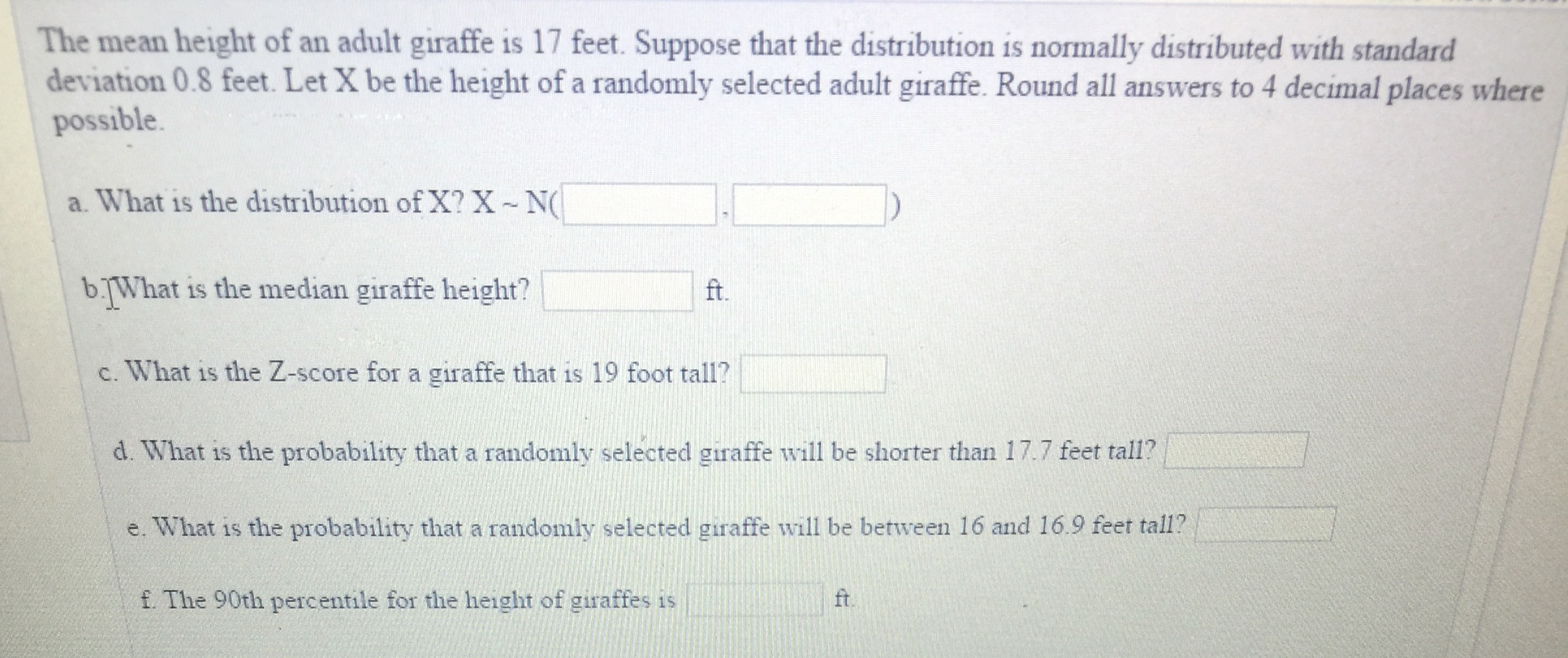# The mean height of an adult giraffe is 17 feet. Suppose that the distribution is normally distributed with standarddeviation 0.8 feet. Let X be the height of a randomly selected adult giraffe. Round all answers to 4 decimal places wherepossiblea. What is the distribution of X? X Nb.What is the median giraffe height?ft.c. What is the Z-score for a giraffe that is 19 foot tall?d. What is the probability that a randomly selected giraffe will be shorter than 17.7 feet tall?e. What is the probability that a randomly selected giraffe will be between 16 and 16.9 feet tall?f. The 90th percentile for the height of giraffes isft.

Question
663 viewshelp_outlineImage TranscriptioncloseThe mean height of an adult giraffe is 17 feet. Suppose that the distribution is normally distributed with standard deviation 0.8 feet. Let X be the height of a randomly selected adult giraffe. Round all answers to 4 decimal places where possible a. What is the distribution of X? X N b.What is the median giraffe height? ft. c. What is the Z-score for a giraffe that is 19 foot tall? d. What is the probability that a randomly selected giraffe will be shorter than 17.7 feet tall? e. What is the probability that a randomly selected giraffe will be between 16 and 16.9 feet tall? f. The 90th percentile for the height of giraffes is ft. fullscreen
check_circle

Step 1

Note: Since we are entitled to answer up to 3 sub-parts, we’ll answer the first 3 as you have not mentioned the subparts you need help with. Please resubmit the question and specify the other subparts you’d like to get answered

In this question, it has been given that X is a random variable which represents the height of giraffe

Step 2

Part a)

X follows the normal distribution with mean equal to 17 feet and standard deviation equal to 0.8 feet.

This is clearly mentioned in the question itself that X follows the normal distribution and also the value of mean and standard deviation is also mentioned in question.

Step 3

Part b)

What is the median giraffe height?

The median giraffe height is 17 feet because X which is a random variable which represents the height of giraffe follows the normal distrib...

### Want to see the full answer?

See Solution

#### Want to see this answer and more?

Solutions are written by subject experts who are available 24/7. Questions are typically answered within 1 hour.*

See Solution
*Response times may vary by subject and question.
Tagged in

### Statistics